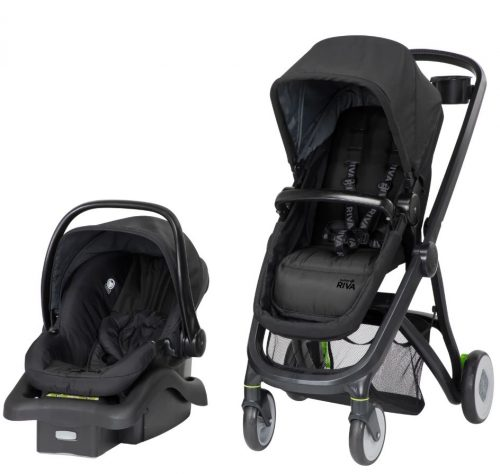# Giveaway Testsome text about the prize here, some text about the prize here, some text about the prize here, some text about the prize here, some text about the prize here, some text about the prize here, some text about the prize here, some text about the prize here, some text about the prize here, some text about the prize here

some text about the prize here, some text about the prize here

some text about the prize here, some text about the prize here